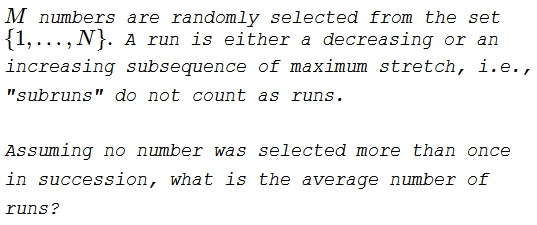# Average Number of Runs in a Sequence of Random Numbers

### Problem### Solution 1

A run can start at any one term of the sequence but the last one and there is always a run that starts at the very first term. If $p_k$ is the probability of a run starting in position $k$ then, clearly $p_1=1$ while $p_M=0.$ The average number of runs is simply $\displaystyle R=\sum_{k=1}^{M}p_k,$ or $\displaystyle R=1+\sum_{k=2}^{M-1}p_k.$

Let $n_k$ be the number in the $k^{th}$ position. The probability $p_k$ of a run starting at position $k$ is complementary to the event that, in magnitude, $n_k$ is between its two neighbors: either $n_{k-1}\lt n_k\lt n_{k+1}$ or $n_{k-1}\gt n_k\gt n_{k+1}.$ The latter two events are equiprobable and each comes with the probability of $\displaystyle \frac{{N\choose 3}}{N(N-1)^2}$ because there are $\displaystyle {N\choose 3}$ ways to select three numbers out of $N$ and only one way to order them in a given order, either increasing or decreasing, $N$ ways to choose the first number and $N-1$ ways to choose each of the other two.

Thus, the probability of a run starting at the $k^{th}$ position is

\displaystyle\begin{align} p_k&=1-2\frac{{N\choose 3}}{N(N-1)^2}=1-2\frac{N(N-1)(N-2)}{3!\cdot N(N-1)^2}\\ &=1-\frac{N-2}{3(N-1)}\\ &=\frac{2N-1}{3(N-1)}. \end{align}

The average number of runs then is simply

$\displaystyle R=1+\sum_{k=2}^{M-1}p_k=1+(M-2)\frac{2N-1}{3(N-1)}.$

### Solution 2

An alternation is a transition from an up run to a down run. The number of runs is one plus the number of alternations.

$\displaystyle R(x_1,\dots, x_M):=1+\sum_{i=2}^{M-1} \left[x_i\lt\min\{x_{i-1},x_{i+1}\}\right]+\sum_{i=2}^{M-1} \left[x_i\gt \max\{x_{i-1},x_{i+1}\}\right]$

\displaystyle \begin{align} E[R(x_1,\dots, x_M)] &= 1+\sum_{i=2}^{M-1} Pr\left(x_i\lt\min\{x_{i-1},x_{i+1}\}\right)+\sum_{i=2}^{M-1} Pr\left(x_i\gt\max\{x_{i-1},x_{i+1}\}\right) \end{align}

Note 1:

\displaystyle \begin{align} Pr\left(x_i<\min\{x_{i-1},x_{i+1}\}\right)& =\sum_{k=1}^{N-1}Pr(\min\{x_{i-1},x_{i+1}\}\gt k\ |x_i=k)Pr(x_i=k)\\ &=\sum_{k=1}^{N-1}\left(\frac{N-k}{N-1}\right)^2\frac{1}{N}\\ &=\frac{2N-1}{6N-6} \end{align}

where the second line follows from conditional independence.

Note 2:

\displaystyle \begin{align} Pr\left(x_i>\max\{x_{i-1},x_{i+1}\}\right)& =\sum_{k=2}^{N}Pr(\max\{x_{i-1},x_{i+1}\}\lt k\ |x_i=k)Pr(x_i=k)\\ &=\sum_{k=2}^{N}\left(\frac{k-1}{N-1}\right)^2\frac{1}{N}\\ &=\frac{2N-1}{6N-6} \end{align}

Combining together:

\displaystyle \begin{align} E[R(x_1,\dots, x_M)] &= 1+2(M-2)\frac{2N-1}{6N-6}\\ &=1+(M-2)\frac{2N-1}{3(N-1)} \end{align}

### Remark

For $N=2,$ the formula reduces to $\displaystyle 1+(M-2)\frac{2\cdot 2-1}{3(2-1)}=M-1,$ implying that the experiment is only possible if $M\le N.$ There are just two possible outcomes: $1,2,\ldots,N$ and $N,N-1,\ldots,1.$ This is due to the stipulation that no two successive selections may be equal. If the stipulation is removed, then the problem becomes equivalent to finding the average length of the runs in coin tossing, which, as we know, equals $\displaystyle \frac{M+1}{2}.$

### Acknowledgment

This is an extension of a problem proposed by the USA for the 1987 IMO. The problem itself was discussed in R. Honsberger's From Erdös To Kiev (MAA, 1996, 29-33). Solution 2 is by Joshua B. Miller.